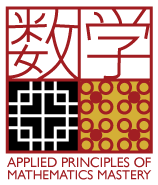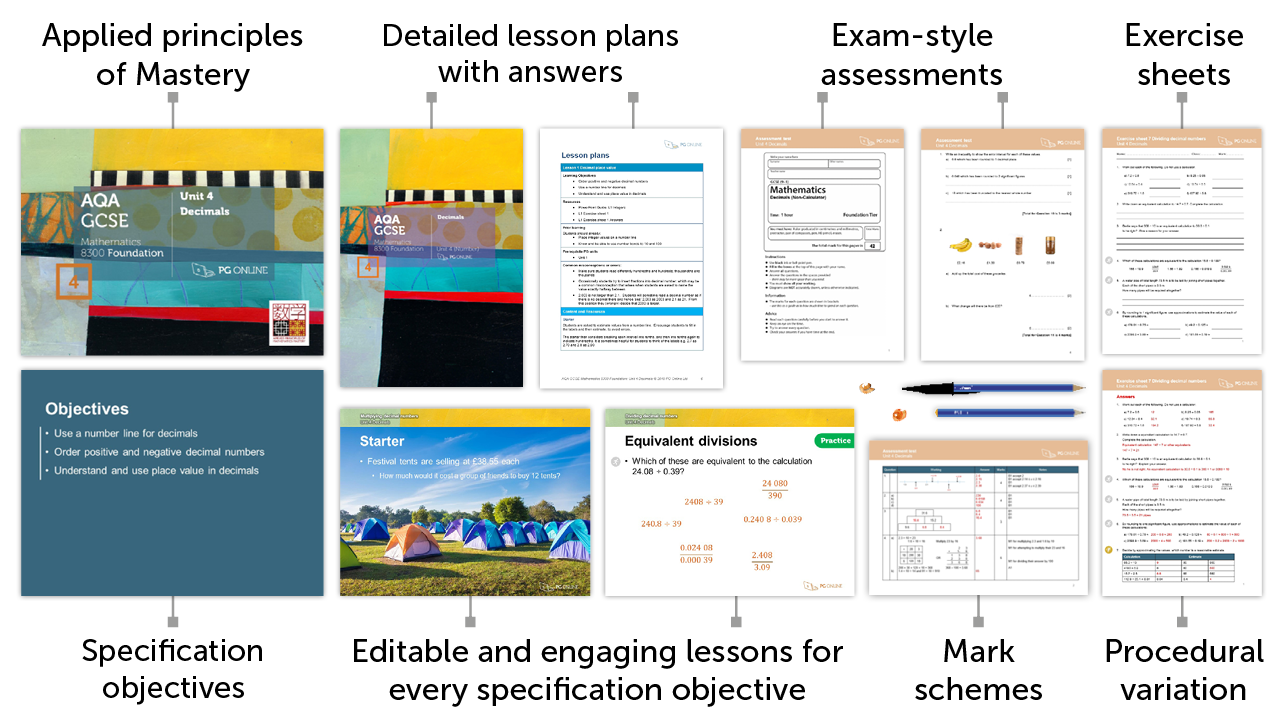# Mastery for GCSE AQA

### FREETry FREE today+

4

## Inside the Unit...### Areas Covered

• Lesson 1: Decimal place value
• Lesson 2: Calculating with decimal numbers
• Lesson 3: Rounding and decimal places
• Lesson 4: Significant figures
• Lesson 5: Error intervals
• Lesson 6: Multiplying decimal numbers
• Lesson 7: Dividing decimal numbers
• Unit assessment

There are 7 exercise sheets and an examination-style assessment test, each with answers included in this unit.

## Mathematics Mastery

Our resources apply the principles of Mastery throughout, creating a rich and deep learning experience. Find out more about Mastery with our quick guide.## Summary

This FREE unit builds on the work completed in Unit 1 with integers, by extending into decimal values. Initially place value is explored and decimal numbers compared and ordered. The four mathematical operations are considered in the context of decimals and consolidated through intelligent practice. The impact of multiplying and dividing by powers of 10 is explored and place value is used to solve reasoning problems. Techniques with rounding numbers, significant figures and decimal places are developed and the use of approximations to support problems is considered. The work on approximations continues by developing the use of inequalities to express error intervals for rounded and truncated numbers. Contextualised problems help develop a range of written methods to multiply and divide decimal numbers.

## What's included in the toolkit?The GCSE units have been written to satisfy the specification for the AQA 8300 Maths GCSE. Each unit contains:

• PowerPoint slides for each component
• Detailed lesson plans
• Learning objectives and outcomes
• Worksheets and homework activities with answers
• End-of-unit, exam-style assessment test with answers
• Other material and links to online resources

## What people say...

Thank you for your excellent resources - the amount of time the resources save is brilliant!

Practice questions are set in context where appropriate so students see the relevance of the mathematics they are learning.

Heather Davis. Professional Development Lead, NCETM

## Inside the Unit...

• ### Areas Covered

• Lesson 1: Decimal place value
• Lesson 2: Calculating with decimal numbers
• Lesson 3: Rounding and decimal places
• Lesson 4: Significant figures
• Lesson 5: Error intervals
• Lesson 6: Multiplying decimal numbers
• Lesson 7: Dividing decimal numbers
• Unit assessment

There are 7 exercise sheets and an examination-style assessment test, each with answers included in this unit.

## Mathematics Mastery

Our resources apply the principles of Mastery throughout, creating a rich and deep learning experience. Find out more about Mastery with our quick guide.• ### How to order

1. Add individual units to a draft order or download a blank order form below to complete manually

2. Using a draft order you can either:

2. Email us your complete order
3. Create a PDF
(to fax or email at a later date)

• ### Unit Pricing and Discounts

Each unit is individually priced for LIFE. Please view each unit for detail.

Discounts are cumulative based on the total of units licenced to your department.

All prices subject to further discounts and VAT.

There are no annual renewal fees.

 2-5 units 10% discount 6-11 units 15% discount 12-23 units 20% discount 24+ units 25% discount